## GATE 2003

 Question 1

Consider the following C function.

```float f(float x, int y)
{
float p, s; int i;
for (s=1, p=1, i=1; i < y; i ++)
{
p*= x/i;
s+=p;
}
return s;
}  ```

For large values of y, the return value of the function f best approximates

 A Xy B ex C ln(1+x) D Xx
Algorithms        Identify-Function
Question 1 Explanation:
P = P * (x/i)
S = S+P
Iteration 1:
P=1; i=1; S=1
P=x
S = 1+x
Iteration 2:
P=x; S = 1+x; i=2
P = x * x/2 = x2/2
Iteration 3:
P = x2/2; S = 1+x+x2/2; i=3
P = (x2/2)(x/3) = x3/6
S = 1 + x + x2/2 + x3/6
Continue upto n
Then f( ) returns
:
S = 1 + x/1 + x2/2⋅1 + x3/3⋅2 + ...
= 1 + x/1! + x2/2! + x3/3! + ... + xn/n!
= ex
 Question 2

Assume the following C variable declaration

`int *A , B; `

Of the following expressions

`I. A     II. A     III. B     IV. B  `

which will not give compile-time errors if used as left hand sides of assignment statements in a C program?

 A I, II, and IV only B II, III, and IV only C II and IV only D IV only
Data-Structures       Arrays
Question 2 Explanation:
i) A can be consider as a pointer and this will not give any compile-time error.
ii) A This results an integer, no error will come.
iii) B is a base address of an array. This will not be changed it will result a compile time error.
iv) B This also results an integer. No error will come.
 Question 3

Let P(E) denote the probability of the event E. Given P(A) = 1, P(B) = 1/2, the values of P(A|B) and P(B|A) respectively are

 A 1/4, 1/2 B 1/2, 1/4 C 1/2, 1 D 1, 1/2
Engineering-Mathematics       Probability
Question 3 Explanation:
P(A)=1, P(B)=1/2
P(A/B) = P(A∩B)/P(B)
= P(A)⋅P(B)/P(B) (consider P(A), P(B) are two independent events)
= 1
P(B/A) = P(B∩A)/P(A)
= P(B)⋅P(A)/P(A)
= 1/2
 Question 4

Let A be a sequence of 8 distinct integers sorted in ascending order. How many distinct pairs of sequences, B and C are there such that (i) each is sorted in ascending order, (ii) B has 5 and C has 3 elements, and (iii) the result of merging B and C gives A?

 A 2 B 30 C 56 D 256
Algorithms Sorting
Question 4 Explanation:
A can have sequence of 8 distinct integers which are sorted in ascending order.
→ If we are pick 3 elements from 8 sequence integers then remaining 5 elements are already in ascending order. After merging these elements then it gives A.
→ No. of possibilities of choosing 8 elements from total of 8 = 8C3
= 8!/3!5!
= 8 * 7
= 56
 Question 5

n couples are invited to a party with the condition that every husband should be accompanied by his wife. However, a wife need not be accompanied by her husband. The number of different gatherings possible at the party is

 ABCD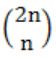Engineering-Mathematics       Combinatorics
Question 5 Explanation:
The possibilities to attend party is
i) Both husband and wife comes
ii) Only wife comes
iii) Both are not come
The no. of different gatherings possible at party is
= 3 * 3 * 3 * 3 * ... n times
= 3n
 Question 6

Let T(n) be the number of different binary search trees on n distinct elements. Then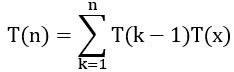, where x is

 A n – k + 1 B n – k C n – k – 1 D n – k – 2
Data-Structures       Binary-Search-Tree
Question 6 Explanation:
A binary search tree consists of n distinct elements. Let consider on left subtree, it consists of (k-1) elements. Then right subtree consists of (n-k) elements. From this we c an write recursive function as T(k-1)*(n-k) i.e.,Question 7

Consider the set ∑* of all strings over the alphabet ∑ = {0, 1}. ∑* with the concatenation operator for strings

 A does not form a group B forms a non-commutative group C does not have a right identity element D forms a group if the empty string is removed from Σ*
Engineering-Mathematics       Sets-And-Relation
Question 7 Explanation:
In the concatenation '∊' is the identity element. And given one is not a group because no element has inverse element.
→ To perform concatenation with the given set can result a Monoid and it follows the property of closure, associativity and consists of identity element.
 Question 8

Let G be an arbitrary graph with n nodes and k components. If a vertex is removed from G, the number of components in the resultant graph must necessarily lie between

 A k and n B k – 1 and k + 1 C k – 1 and n – 1 D k + 1 and n – k
Engineering-Mathematics       Graph-Theory
Question 8 Explanation:
While a vertex is removed from a graph then that can be itself be forms a new component. The minimum number of components is k-1.
If a vertex is removed then it results that all the components are also be disconnected. So removal can create (n-1) components.
 Question 9

Assuming all numbers are in 2's complement representation, which of the following numbers is divisible by 11111011?

 A 11100111 B 11100100 C 11010111 D 11011011
Digital-Logic-Design       Number-Systems
Question 9 Explanation:
Given: Binary numbers = 11111011
MSB bit is '1' then all numbers are negative
1's complement = 00000100
2's complement = 00000100 + 00000001 = 00000101 = -5
(A) 11100111 - (-25)10
(B) 11100100 - (-28)10
(C) 11010111 - (-41)10
(D) 11011011 - (-37)10
Answer: Option A (-25 is divisible by -5)
 Question 10

For a pipelined CPU with a single ALU, consider the following situations

```I. The j + 1-st instruction uses the result of the j-th instruction as an operand
II. The execution of a conditional jump instruction
III. The j-th and j + 1-st instructions require the ALU at the same time ```

Which of the above can cause a hazard?

 A I and II only B II and III only C III only D All the three
Computer-Organization       Pipelining
Question 10 Explanation:
I is belongs to the Data hazard.
II is belongs to the Control hazard.
III is belongs to the Structural hazard.
→ Hazards are the problems with the instruction pipeline in CPU micro architectures.
 Question 11

Consider an array multiplier for multiplying two n bit numbers. If each gate in the circuit has a unit delay, the total delay of the multiplier is

 A Θ (1) B Θ (log n) C Θ (n) D Θ (n2)
Digital-Logic-Design       Multiplexer
Question 11 Explanation:
Each bit in Multiplier is ANDed with a bit in Multiplicand which produce n n-bit numbers. The multiplication takes n units of time. The n n-bit numbers are added by using (n-1) n-bit adders. The time taken by (n-1) n-bit adders is k*(n-1) units.
The total time is n+kn-k = Θ(n)
 Question 12

Ram and Shyam have been asked to show that a certain problem Π is NP-complete. Ram shows a polynomial time reduction from the 3-SAT problem to Π, and Shyam shows a polynomial time reduction from Π to 3-SAT. Which of the following can be inferred from these reductions?

 A Π is NP-hard but not NP-complete B Π is in NP, but is not NP-complete C Π is NP-complete D Π is neither NP-hard, nor in NP
Algorithms        P-NP
Question 12 Explanation:
Note: As per Present syllabus, it is not required.
 Question 13

Nobody knows yet if P = NP. Consider the language L defined as follows: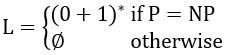Which of the following statements is true?

 A L is recursive B L is recursively enumerable but not recursive C L is not recursively enumerable D Whether L is recursive or not will be known after we find out if P = NP
Theory-of-Computation       Recursive-Enumerable-Languages
Question 13 Explanation:
Here, we have two possibilities, whether
P = NP (or) P != NP
→ If P=NP then L=(0+1)* which is regular, then it is recursive.
→ If P!=NP then L becomes ɸ which is also regular, then it is recursive.
So, finally L is recursive.
 Question 14

The regular expression 0*(10*)* denotes the same set as

 A (1*0)*1* B 0+(0+10)* C (0+1)*10(0+1)* D None of the above
Theory-of-Computation       Regular-Expressions
Question 14 Explanation:
Both (A) and the given expression generates all strings over Σ.
Option (B) and (C) doesn't generate 11.
 Question 15

If the strings of a language L can be effectively enumerated in lexicographic (i.e., alphabetic) order, which of the following statements is true?

 A L is necessarily finite B L is regular but not necessarily finite C L is context free but not necessarily regular D L is recursive but not necessarily context free
Theory-of-Computation       Identify-Class-Language
Question 15 Explanation:
The given language L is to be recursively enumerable. The TM which accepts the language which is in lexicographic order. If the language is not in lexicographic order which is not accepted by TM.
The give 'L' is recursive but not necessarily context free.
 Question 16

Which of the following suffices to convert an arbitrary CFG to an LL(1) grammar?

 A Removing left recursion alone B Factoring the grammar alone C Removing left recursion and factoring the grammar D None of the above
Compiler-Design       Grammar
Question 16 Explanation:
Left recursion removing (or) factoring the given grammar are not sufficient to convert an arbitrary CFG to an LL(1) grammar.
To convert an arbitrary CFG to an LL(1) grammar we need to remove the left recursion and as well as left factoring without that we cannot convert.
 Question 17

Assume that the SLR parser for a grammar G has n1 states and the LALR parser for G has n2 states. The relationship between n1 and n2 is:

 A n1 is necessarily less than n2 B n1 is necessarily equal to n2 C n1 is necessarily greater than n2 D None of the above
Compiler-Design       Parsers
Question 17 Explanation:
No. of states in SLR and LALR are equal and no. of states in SLR and LALR are less than or equal to LR(1).
 Question 18

In a bottom-up evaluation of a syntax directed definition, inherited attributes can

 A always be evaluated B be evaluated only if the definition is L-attributed C be evaluated only if the definition has synthesized attributes D never be evaluated
Compiler-Design       Syntax-Directed-Translation
Question 18 Explanation:
L-Attributed grammar can able to inherits either inherited attributes (or) synthesized attributes.
L-Attributed definitions are a class of syntax directed definitions whose attributes can be evaluated by a single traversal of the parse-tree.
 Question 19

Suppose the numbers 7, 5, 1, 8, 3, 6, 0, 9, 4, 2 are inserted in that order into an initially empty binary search tree. The binary search tree uses the usual ordering on natural numbers. What is the in-order traversal sequence of the resultant tree?

 A 7 5 1 0 3 2 4 6 8 9 B 0 2 4 3 1 6 5 9 8 7 C 0 1 2 3 4 5 6 7 8 9 D 9 8 6 4 2 3 0 1 5 7
Data-Structures       Binary-Search-Tree
Question 19 Explanation:Inorder: 0 1 2 3 4 5 6 7 8 9
 Question 20

Consider the following three claims

```I. (n + k)m = Θ(nm), where k and m are constants
II. 2n+1 = O(2n)
III. 22n+1 = O(2n) ```

Which of these claims are correct?

 A I and II B I and III C II and III D I, II, and III
Algorithms        Time-Complexity
Question 20 Explanation:
I) (n+k)m = Θ(nm)
Which is true by considering leading ordered term present in polynomial expression.
II) 2n+1 = Θ(nm) → True2n×2n can't be written as Θ(2n)
So, this is False.
 Question 21

Consider the following graphAmong the following sequences:

`(I) a b e g h f    (II) a b f e h g    (III) a b f h g e    (IV) a f g h b e `

Which are depth first traversals of the above graph?

 A I, II and IV only B I and IV only C II, III and IV only D I, III and IV only
Data-Structures       Graphs
Question 21 Explanation:
I) a → b → e → g → h → f (✔️)
II) a → b → f → e (✖️)
III) a → b → f → h → g → e (✔️)
IV) a → f → g → h → b → e (✔️)
 Question 22

The usual Θ(n2) implementation of Insertion Sort to sort an array uses linear search to identify the position where an element is to be inserted into the already sorted part of the array. If, instead, we use binary search to identify the position, the worst case running time will

 A remain Θ(n2) B become Θ(n (log n)2) C become Θ(n log n) D become Θ(n)
Algorithms        Time-Complexity
Question 22 Explanation:
While using Insertion sort to sort array by using linear search then time complexity = Θ(n2)
Instead, linear search use binary search then (log n) will be the worst case time complexity of binary search and performing n swaps to place an element in right position for the corresponding n elements
i.e., n×(logn+n)
Θ((n×logn)+n2)
Θ(n2)
Remains same.
 Question 23

In a heap with n elements with the smallest element at the root, the 7th smallest element can be found in time

 A Θ(n log n) B Θ(n) C Θ(log n) D Θ(1)
Data-Structures       Heap-Tree
Question 23 Explanation:
The 7th smallest elements can be present in any of 7 levels. Then total possible elements can be present is seven levels is
1 + 2 + 4 + 6 + 8 + 16 + 32
Which is constant then we can find the 7th smallest element in Θ(1) time.
 Question 24

Which of the following statements is FALSE?

 A In statically typed languages, each variable in a program has a fixed type B In un-typed languages, values do not have any types C In dynamically typed languages, variables have no types D In all statically typed languages, each variable in a program is associated with values of only a single type during the execution of the program
Compiler-Design       Run-Time-Environments
Question 24 Explanation:
Dynamic typed languages are those languages in which variable must necessarily be defined before they are used. Then dynamic typed languages have types.
 Question 25

Using a larger block size in a fixed block size file system leads to:

 A better disk throughput but poorer disk space utilization B better disk throughput and better disk space utilization C poorer disk throughput but better disk space utilization D poorer disk throughput and poorer disk space utilization
Computer-Organization       Disk-Scheduling
Question 25 Explanation:
While using a larger block size means that contains less number of blocks then that results better throughput. This can be implemented in a fixed block size then the space utilization is not upto the mark. So the statement results better disk throughput but poorer disk space utilization.
 Question 26

In a system with 32 bit virtual addresses and 1 KB page size, use of one-level page tables for virtual to physical address translation is not practical because of

 A the large amount of internal fragmentation B the large amount of external fragmentation C the large memory overhead in maintaining page tables D the large computation overhead in the translation process
Operating-Systems       Virtual Memory
Question 26 Explanation:
Page size = 1KB
Virtual address = 32 bit = 232
No. of page level entries = 232 / 210
= 222
= 4M (Too large size)
 Question 27

Which of the following assertions is FALSE about the Internet Protocol (IP)?

 A It is possible for a computer to have multiple IP addresses B IP packets from the same source to the same destination can take different routes in the network C IP ensures that a packet is discarded if it is unable to reach its destination within a given number of hops D The packet source cannot set the route of an outgoing packets; the route is determined only by the routing tables in the routers on the way
Computer-Networks       IPv4
Question 27 Explanation:
Because in strict source routing or loose source routing path is set by the source not by router and main task of router is to check outgoing path with the help of forwarding table inside it.
 Question 28

Which of the following functionalities must be implemented by a transport protocol over and above the network protocol?

 A Recovery from packet losses B Detection of duplicate packets C Packet delivery in the correct order D End to end connectivity
Computer-Networks       Transport Layer Protocol
Question 28 Explanation:
End to end connectivity is the required functionality provided by Transport protocol.
 Question 29

Which of the following scenarios may lead to an irrecoverable error in a database system?

 A A transaction writes a data item after it is read by an uncommitted transaction B A transaction reads a data item after it is read by an uncommitted transaction C A transaction reads a data item after it is written by a committed transaction D A transaction reads a data item after it is written by an uncommitted transaction
Database-Management-System       Transactions
Question 29 Explanation:
Irrecoverable error occurs when a transaction reads a data item after it is written by uncommitted transaction.
 Question 30

Consider the following SQL query

```   select distinct al, a2,........., an
from rl, r2,........, rm
where P ```

For an arbitrary predicate P, this query is equivalent to which of the following relational algebra expressions?

 ABCDDatabase-Management-System       SQL
Question 30 Explanation:
If we want to get distinct elements then we need to perform cross product in between the relations r1, r2, .... rm.
 Question 31

Let (S, ≤) be a partial order with two minimal elements a and b, and a maximum element c. Let P: S → {True, False} be a predicate defined on S. Suppose that P(a) = True, P(b) = False and P(x) ⇒ P(y) for all x, y ∈ S satisfying x ≤ y, where ⇒ stands for logical implication. Which of the following statements CANNOT be true?

 A P(x) = True for all x ∈ S such that x ≠ b B P(x) = False for all x ∈ S such that x ≠ a and x ≠ c C P(x) = False for all x ∈ S such that b ≤ x and x ≠ c D P(x) = False for all x ∈ S such that a ≤ x and b ≤ x
Engineering-Mathematics       Sets-And Relation
Question 31 Explanation:
c is the maximum element.
a or b the minimal element in set.
P(a) = True for all x ∈ S such that a ≤ x and b ≤ x.
Option D is False.
 Question 32

Which of the following is a valid first order formula? (Here α and β are first order formulae with x as their only free variable)

 A ((∀x)[α] ⇒ (∀x)[β]) ⇒ (∀x)[α⇒β] B (∀x)[α] ⇒ (∃x)[α ∧ β] C ((∀x)[α ∨ β] ⇒ (∃x)[α] ⇒ (∀x)[α] D (∀x)[α ⇒ β] ⇒ ((∀x)[α] ⇒ (∀x)[β])
Engineering-Mathematics       Propositional-Logic
Question 32 Explanation:
Option D is valid.
Here, α, β are holding values of x. Then and RHS saying that α holding the value of x and β is holding value of x.
Then LHS ⇒ RHS.
 Question 33

Consider the following formula a and its two interpretations I1 and I2

```α: (∀x)[Px ⇔ (∀y)[Qxy ⇔ ¬Qyy]] ⇒ (∀x)[¬Px]
I1: Domain: the set of natural numbers
Px ≡ 'x is a prime number'
Qxy ≡ 'y divides x'
I2: same as I1 except that Px = 'x is a composite number'.```

Which of the following statements is true?

 A I1 satisfies α, I2 does not B I2 satisfies α, I1 does not C Neither I2 nor I1 satisfies α D Both I1 and I2 satisfy α
Engineering-Mathematics       Propositional-Logic
Question 33 Explanation:
Given that:
(∀x)[Px ⇔ (∀y)[Qxy ⇔ ¬Qyy]] ⇒(∀x)[¬Px]
Qyy is always true, because y divide y, then ¬Qyy is false.
∀x[(P(x) ⇔ ∀y [Qxy ⇔ False]]
∀y [Qxy ⇔ False] can be written as ∀y[¬axy]
⇒(∀x)[P(x) ⇔ ∀y[¬Qxy]]
Here, ¬Qxy says that y doesnot divides x, which is not always be true.
For example, if x=y then it is false then ∀y[¬Qxy] is not true for all values of y.
⇒(∀x)[P(x) ⇔ False]
⇒(∀x)[¬P(x) = RHS]
LHS = RHS
⇒ Irrespective of x, whether x is prime of composite number I1 and I2 satisfies α.
 Question 34

m identical balls are to be placed in n distinct bags. You are given that m ≥ kn, where, k is a natural number ≥1. In how many ways can the balls be placed in the bags if each bag must contain at least k balls?

 ABC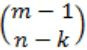DEngineering-Mathematics       Combinatorics
Question 34 Explanation:
Since we want at least k balls in each bag, so first we put kn balls into bags, k balls in each bag. Now we are left with m - kn balls, and we have to put them into n bags such that each bag may receive 0 or more balls. So applying theorem 2 of stars and bars with m - nk stars and n bars, we get number of ways to be. So option (B) is correct.
 Question 35

Consider the following recurrence relation

```T(1) = 1
T(n+1) = T(n) + ⌊√n+1⌋ for all n≥1 ```

The value of T(m2) for m ≥ 1 is

 A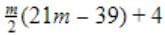B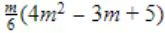C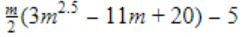DAlgorithms        Recurrence
Question 35 Explanation: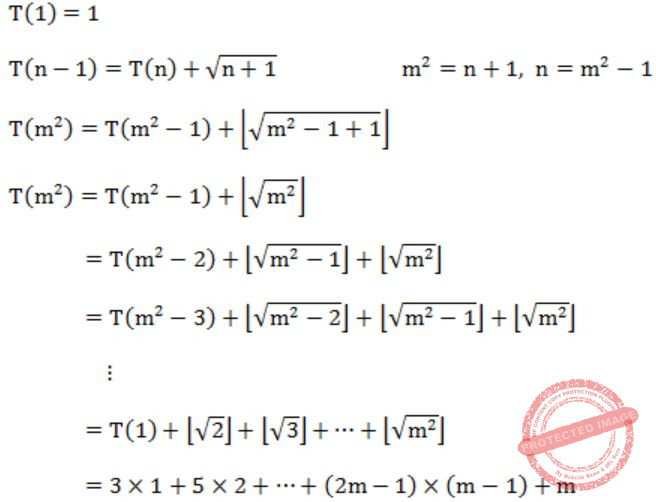Considering floor value for square root of numbers.
Successive root number of numbers are in the series 3, 5, 7, ... like 3 numbers from 1... 4, 5 numbers 5-9 and so on.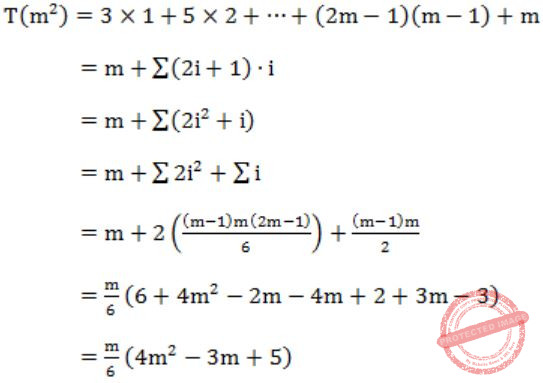Question 36

How many perfect matching are there in a complete graph of 6 vertices ?

 A 15 B 24 C 30 D 60
Engineering-Mathematics       Graph-Theory
Question 36 Explanation:
We have formula to find no. of perfect matching in complete graphs of 2n vertices,
(2n)!/n!×2n
Given, 2n = 6 ⇒ n = 3
So, finally, 6!/3!×23 = 15
 Question 37

Let f : A → B be an injective (one-to-one) function. Define g: 2A→2B as: g(C) = {f(x)|x ∈ C}, for all subsets C of A.
Define h: 2B → 2A as: h(D) = {x|x ∈ A, f(x) ∈ D}, for all subsets D of B.

Which of the following statements is always true?

 A g(h(D)) ⊆ D B g(h(D)) ⊇ D C g(h(D)) ∩ D = ɸ D g(h(D)) ∩ (B—D) ≠ ɸ
Engineering-Mathematics       Sets and Functions
Question 37 Explanation:
f: A→B be an injective (one-to-one)
→ g: 2A→2B be also one to one function and g(C) = f(x)|x∈C}, for all subsets C of A.
The range of this function is n(2A).
→ h: 2B→2A it is not a one to one function and given h(D) = {x|x∈A, f(x)∈D}, for all subsets D of B.
The range of this function is also n(2A).
→ The function g(h(D)) also have the range n(2A) that implies n(A)≤n(B), i.e., n(2A) is less than n(2B).
Then this result is g(h(D)) ⊆ D.
 Question 38

Consider the set {a, b, c} with binary operators + and × defined as follows:

```+	a	b	c		×	a	b	c
a	b	a	c		a	a	b	c
b	a	b	c		b	b	c	a
c	a	c	b		c	c	c	b ```

For example, a + c = c, c + a = a, c × b = c and b × c = a. Given the following set of equations:

```(a × x) + (a × y) = c
(b × x) + (c × y) = c ```

The number of solution(s) (i.e., pair(s) (x, y)) that satisfy the equations is:

 A 0 B 1 C 2 D 3
Engineering-Mathematics       Sets-And-Relation
Question 38 Explanation:
Total possible values = 32 = 9 i.e., (a,a), (b,b), (c,c), (a,b), (a,c), (b,a), (b,c), (c,a), (c,b)
In those (x, y) = (b,c) & (c,b) are the possible solution for the corresponding equations.
(x, y) = (b,c) ⇒ (a*b)+(a*c) ⇒ (b*b)+(c*c)
⇒ (b) + (c) ⇒ c + b
⇒ c (✔️) ⇒ c (✔️)
(x,y) = (c,b) ⇒ (a*c)+(a*b) ⇒ (b*c)+(c*b)
⇒ c+b ⇒ a+c
⇒ c (✔️) ⇒ c (✔️)
 Question 39

Let ∑ = (a, b, c, d, e) be an alphabet. We define an encoding scheme as follows:

`g(a) = 3, g(b) = 5, g(c) = 7, g(d) = 9, g(e) = 11.`

Let pi denote the i-th prime number (p1=2).

For a non-empty string s=a1 ... an, where each ai∈Σ, defineFor a non-empty sequence 〈sj...sn〉 of strings from ∑+, define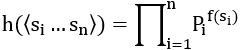Which of the following numbers is the encoding h of a non-empty sequence of strings?

 A 273757 B 283858 C 293959 D 210510710
Engineering-Mathematics       Sets-And-Relation
Question 39 Explanation:
The answers can have only three possibilities of sequences i.e., "a", "a", "a", and there is no multiplies of 7 and 9. So eliminates option A & C.
And f(S) = 23 = 8
So answer is 283858.
 Question 40

A graph G = (V,E) satisfies |E|≤ 3|V|-6. The min-degree of G is defined as. Therefore, min-degree of G cannot be

 A 3 B 4 C 5 D 6
Engineering-Mathematics       Graph-Theory
Question 40 Explanation:
The minimum degree of G = minv∈V {degree(v)}
|E| ≤ 3|v| - 6
Based on handshaking lemma, the minimum degree is (min×|v|)/2
⇒ (min×|v|)/2 ≤ 3|v| - 6
Checking the options lets take min=6
(6×|v|)/2 ≤ 3|v| - 6
0 ≤ -6 (Not satisfied)
And which is inconsistent.
 Question 41

Consider the following system of linear equationNotice that the second and the third columns of the coefficient matrix are linearly dependent. For how many values of α, does this system of equations have infinitely many solutions?

 A 0 B 1 C 2 D infinitely many
Engineering-Mathematics       Linear-Algebra
Question 41 Explanation: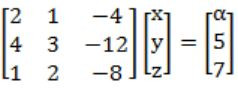This is in the form AX = B⇒ R(AB) < n [If we want infinitely many solution]
then -1+5α = 0
5α = 1
α = 1/5 There is only one value of α. System can have infinitely many solutions.
 Question 42

A piecewise linear function f(x) is plotted using thick solid lines in the figure below (the plot is drawn to scale).If we use the Newton-Raphson method to find the roots of f(x) = 0 using x0, x1 and x2 respectively as initial guesses, the roots obtained would be

 A 1.3, 0.6, and 0.6 respectively B 0.6, 0.6, and 1.3 respectively C 1.3, 1.3, and 0.6 respectively D 1.3, 0.6, and 1.3 respectively
Engineering-Mathematics       Newton-Raphson-Method
Question 42 Explanation:
Note: Out of syllabus.
 Question 43

The following is a scheme for floating point number representation using 16 bits.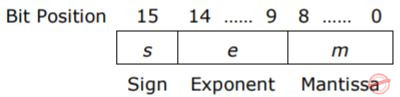Let s, e, and m be the numbers represented in binary in the sign, exponent, and mantissa fields respectively. Then the floating point number represented is:What is the maximum difference between two successive real numbers representable in this system?

 A 2-40 B 2-9 C 222 D 231
Digital-Logic-Design       Number-Systems
Question 43 Explanation:
Largest gap will be in between two most largest numbers.
The largest number is 1.111111111× 262-31 = (2−2−9)×231
Second largest number is 1.111111110×262-31 = (2−2-8)×231
Difference = (2−2−9)×231 - (2−2-8)×231
= (2-8−2−9) ×231
= 2−9×231
= 222
 Question 44

A 1-input, 2-output synchronous sequential circuit behaves as follows:

Let zk, nk denote the number of 0's and 1's respectively in initial k bits of the input (zk + nk = k). The circuit outputs 00 until one of the following conditions holds.

```•   zk - nk = 2. In this case, the output at the k-th and all subsequent clock ticks is 10.
•   nk - zk = 2. In this case, the output at the k-th and all subsequent clock ticks is 01. ```

What is the minimum number of states required in the state transition graph of the above circuit?

 A 5 B 6 C 7 D 8
Digital-Logic-Design       Sequential-Circuits
Question 44 Explanation:
Let q is the initial state.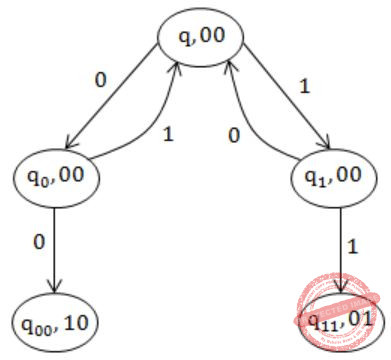q0 ← Number of zeros is one more than number of ones.
q1 ← Number of ones is one more than number of zeros.
q00 ← Number of zeros is two more than number of ones.
q11 ← Number of ones is two more than number of zeros.
 Question 45

The literal count of a boolean expression is the sum of the number of times each literal appears in the expression. For example, the literal count of (xy + xz') is 4. What are the minimum possible literal counts of the product-of-sum and sum-of-product representations respectively of the function given by the following Karnaugh map ? Here, X denotes "don't care"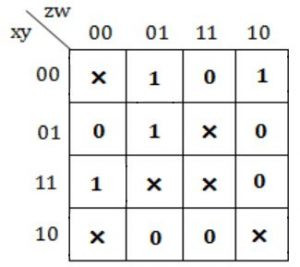A (11, 9) B (9, 13) C (9, 10) D (11, 11)
Digital-Logic-Design       K-Map
Question 45 Explanation:
For SOP,⇒ w'y' + z'wx' + xyz'
Total 8 literals are there.
For POS,⇒ (z' + w')(z' + y')(w' + x')(x + z + w)
Total 9 literals are there.
 Question 46

Consider the ALU shown below.If the operands are in 2's complement representation, which of the following operations can be performed by suitably setting the control lines K and C0 only (+ and - denote addition and subtraction respectively)?

 A A + B, and A – B, but not A + 1 B A + B, and A + 1, but not A – B C A + B, but not A – B or A + 1 D A + B, and A – B, and A + 1
Digital-Logic-Design       Adder
Question 46 Explanation:
The circuits performs
1) A+B when K=0 and C0 = 0. It is binary adder which performs addition of two binary numbers.
2) A - B = A+ B' + 1 when K=1 and C0 = 1 ;
Here XOR gates produce B' if K=1. Since 1⊕b= b'.
"1" in (A+B+1) is coming from C0.
Note: 2's complement of B is (B'+1). 3) A+1 when B=0, K=0, C0= 1.
Increments A.
 Question 47

Consider the following circuit composed of XOR gates and non-inverting buffers.The non-inverting buffers have delays δ1 = 2 ns and δ2 = 4 ns as shown in the figure. Both XOR gates and all wires have zero delay. Assume that all gate inputs, outputs and wires are stable at logic level 0 at time 0. If the following waveform is applied at input A, how many transition(s) (change of logic levels) occur(s) at B during the interval from 0 to 10 ns?A 1 B 2 C 3 D 4
Digital-Logic-Design       Logic-Gates
Question 47 Explanation:⇒ a will always be equal to A.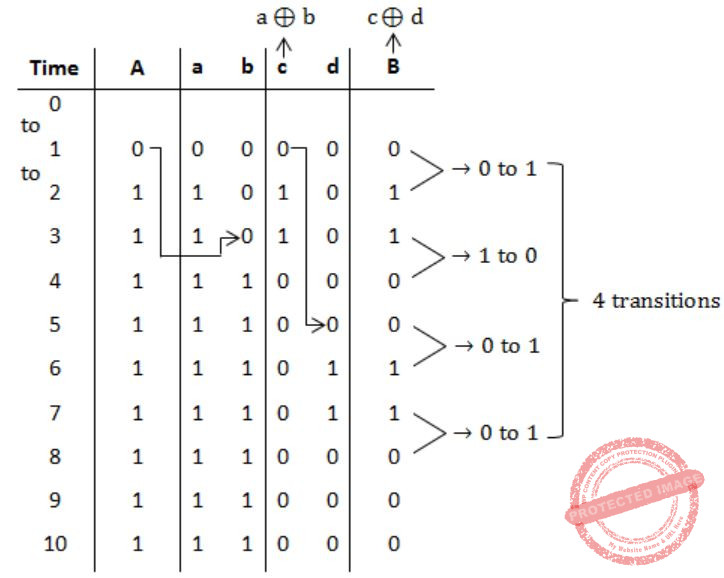Question 48

Consider the following assembly language program for a hypothetical processor. A, B, and C are 8 bit registers. The meanings of various instructions are shown as comments.

```     MOV B, # 0   	;   B ← 0
MOV C, # 8	        ;   C ← 8
Z :  CMP C, # 0	        ;   compare C with 0
JZX	        ;   jump to X if zero flag is set
SUB C, # 1	        ;   C ← C - 1
RRC A, # 1 	;   right rotate A through carry by one bit. Thus:
;   if the initial values of A and the carry flag are a7...a0 and
;   c0 respectively, their values after the execution of this
;   instruction will be c0a7...a1 and a0 respectively.
JC Y	        ;   jump to Y if carry flag is set
JMP Z	        ;   jump to Z
Y :  ADD B, # 1	        ;   B ← B + 1
JMP Z	        ;   jump to Z
X :	```

If the initial value of register A is A0 the value of register B after the program execution will be

 A the number of 0 bits in A0 B the number of 1 bits in A0 C A0 D 8
Computer-Organization       Machine-Instructions
Question 48 Explanation:
B is to be increments when a is moved to carry.
The code is counting the number of 1 bits in A0.
 Question 49

Consider the following assembly language program for a hypothetical processor. A, B, and C are 8 bit registers. The meanings of various instructions are shown as comments.

```     MOV B, # 0   	;   B ← 0
MOV C, # 8	        ;   C ← 8
Z :  CMP C, # 0	        ;   compare C with 0
JZX	        ;   jump to X if zero flag is set
SUB C, # 1	        ;   C ← C - 1
RRC A, # 1 	;   right rotate A through carry by one bit. Thus:
;   if the initial values of A and the carry flag are a7...a0 and
;   c0 respectively, their values after the execution of this
;   instruction will be c0a7...a1 and a0 respectively.
JC Y	        ;   jump to Y if carry flag is set
JMP Z	        ;   jump to Z
Y :  ADD B, # 1	        ;   B ← B + 1
JMP Z	        ;   jump to Z
X :	```

Which of the following instructions when inserted at location X will ensure that the value of register A after program execution is the same as its initial value?

 A RRC A, #1 B NOP ; no operation C LRC A, #1 ; left rotate A through carry flag by one bit D ADD A, #1
Computer-Organization       Machine-Instructions
Question 49 Explanation:
Initially, the 8 bits will be,
a7, a6, a5, a4, a3 , a2, a1, a0
Now right rotate it once,
C0, a7, a6, a5, a4, a3 , a2, a1, now a0 is the new carry.
Now again right rotate it,
a0C0, a7, a6, a5, a4, a3 , a2

So after 8 rotations,
a6, a5, a4, a3 , a2, a1, a0C0 and carry is a7
Now, one more rotation will restore the original value of A0.
Hence, answer is Option (A).
 Question 50

Consider the following deterministic finite state automaton M.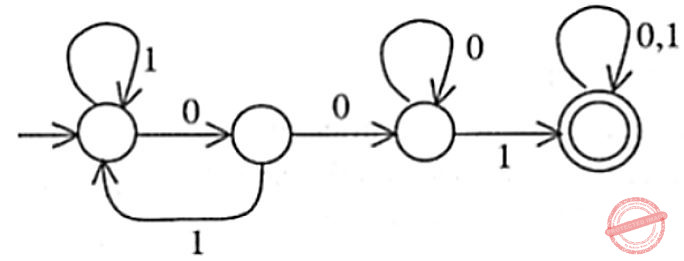Let S denote the set of seven bit binary strings in which the first, the fourth, and the last bits are 1. The number of strings in S that are accepted by M is

 A 1 B 5 C 7 D 8
Theory-of-Computation       Finite-Automata
Question 50 Explanation: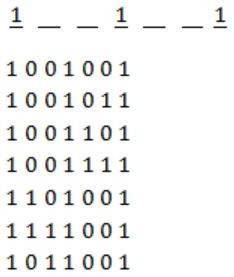There are possible: 7 strings.
 Question 51

Let G = ({S},{a,b},R,S) be a context free grammar where the rule set R is S → aSb | SS | ε Which of the following statements is true?

 A G is not ambiguous B There exist x, y ∈ L(G) such that xy ∉ L(G) C There is a deterministic pushdown automaton that accepts L(G) D We can find a deterministic finite state automaton that accepts L(G)
Theory-of-Computation       Contest-Free-Grammar
Question 51 Explanation:
a) False
We can derive ϵ with more than one parse tree,So ambiguous.
b) False
Let take x=aabb and y=ab then xy=aabbab we can produce it,c) True
Because the language generated is no. of a's = no' of b's. So DPDA exist for this language.
d) Not possible.
Infinite memory needed to count 'a' for no. of 'b'.
 Question 52

Consider two languages L1 and L2 each on the alphabet ∑. Let f: ∑ → ∑ be a polynomial time computable bijection such that (∀ x)[x ∈ L1 iff f(x) ∈ L2]. Further, let f-1 be also polynomial time computable.

Which of the following CANNOT be true?

 A L1 ∈ P and L2 is finite B L1 ∈ NP and L2 ∈ P C L1 is undecidable and L2 is decidable D L1 is recursively enumerable and L2 is recursive
Theory-of-Computation       Decidability-and-Undecidability
Question 52 Explanation:
L1 is polynomial time reducible to L2.
Now if L2 is decidable then L1 should also be decidable. Hence, option (c) is wrong.
 Question 53

A single tape Turing Machine M has two states q0 and q1, of which q0 is the starting state. The tape alphabet of M is {0, 1, B} and its input alphabet is {0, 1}. The symbol B is the blank symbol used to indicate end of an input string. The transition function of M is described in the following table.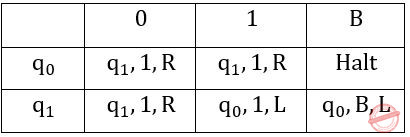The table is interpreted as illustrated below. The entry (q1, 1, R) in row q0 and column 1 signifies that if M is in state q0 and reads 1 on the current tape square, then it writes 1 on the same tape square, moves its tape head one position to the right and transitions to state q1.

Which of the following statements is true about M?

 A M does not halt on any string in (0+1)+ B M does not halt on any string in (00+1)* C M halts on all strings ending in a 0 D M halts on all strings ending in a 1
Theory-of-Computation       Turing Machine
Question 53 Explanation:Try for any string, it will not Halt for any string other than ϵ. Hence, option (A) is correct.
 Question 54

Define languages L0 and L1 as follows:

```L0 = {〈M, w, 0〉 | M halts on w}
L1 = {〈M, w, 1〉 | M does not halts on w} ```

Here 〈M, w, i〉 is a triplet, whose first component. M, is an encoding of a Turing Machine, second component, w, is a string, and third component, i, is a bit.

Let L = L0∪L1. Which of the following is true?

 ABCDTheory-of-Computation       Turing Machine
 Question 55

Consider the NFA M shown below.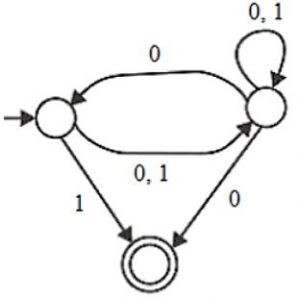Let the language accepted by M be L. Let L1 be the language accepted by the NFA M1, obtained by changing the accepting state of M to a non-accepting state and by changing the non-accepting state of M to accepting states. Which of the following statements is true?

 A L1 = {0,1}* - L B L1 = {0,1}* C L1 ⊆ L D L1 = L
Theory-of-Computation       Finite-Automata
Question 55 Explanation:
As in the question said,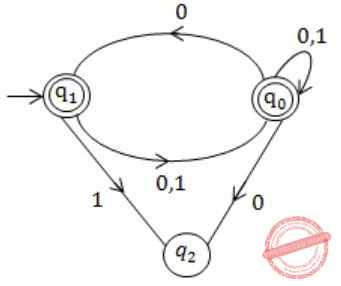As in above NFA language,
L1 is {0,1}*.
 Question 56

Consider the grammar shown below

```S → i E t S S' | a
S' → e S | ε
E → b ```

In the predictive parse table. M, of this grammar, the entries M[S', e] and M[S', \$] respectively are

 A {S'→e S} and {S'→ε} B {S'→e S} and { } C {S'→ε} and {S'→ε} D {S'→e S, S'→ε} and {S'→ε}
Compiler-Design       Parsers
Question 56 Explanation:
First(S) = {1,a}
First(S') = {e,ε}
First(E) = {b}
Follow(S') = {e,\$}
Only when 'First' contains ε, we need to consider FOLLOW for getting the parse table entry.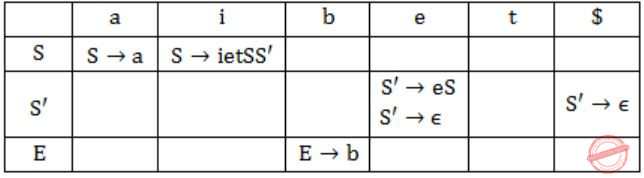Hence, option (D) is correct.
 Question 57

Consider the grammar shown below.

```S → C C
C → c C | d```

The grammar is

 A LL(1) B SLR(1) but not LL(1) C LALR(1) but not SLR(1) D LR(1) but not LALR(1)
Compiler-Design       Parsers
Question 57 Explanation: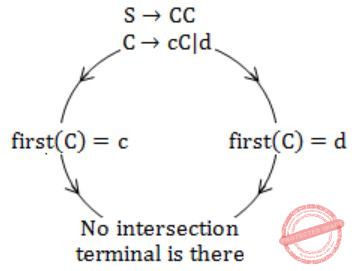Hence, it is LL(1).
 Question 58

Consider the translation scheme shown below.

```S → T R
R → + T {print ('+');} R|ε
T → num {print(num.val);}```

Here num is a token that represents an integer and num.val represents the corresponding integer value. For an input string '9 + 5 + 2', this translation scheme will print

 A 9 + 5 + 2 B 9 5 + 2 + C 9 5 2 + + D + + 9 5 2
Compiler-Design       Syntax-Directed-Translation
Question 58 Explanation:Now traverse the tree and whatever comes first to print, just print it.
Answer will be 9 5 + 2 +.
 Question 59

Consider the syntax directed definition shown below.

```S → id := E           {gen (id.place = E.place;);}
E → E1 + E2           {t = newtemp ( );
gen(t = E1.place + E2.place;);
E.place = t}
E → id                {E.place = id.place;}```

Here, gen is a function that generates the output code, and newtemp is a function that returns the name of a new temporary variable on every call. Assume that ti's are the temporary variable names generated by newtemp. For the statement 'X: = Y + Z', the 3-address code sequence generated by this definition is

 A X = Y + Z B t1 = Y + Z; X = t1 C t1= Y; t2 = t1 + Z; X = t2 D t1 = Y; t2 = Z; t3 = t1 + t2; X = t3
Compiler-Design       Syntax-Directed-Translation
Question 59 Explanation:Question 60

A program consists of two modules executed sequentially. Let f1(t) and f2(t) respectively denote the probability density functions of time taken to execute the two modules. The probability density function of the overall time taken to execute the program is given by:

 A f1(t) + f2(t) BCD max{f1(t), f2(t)}
Engineering-Mathematics       Probability
Question 60 Explanation:
f1(t) and f2(t) are executed sequentially.
→ They representing the probability density functions of time taken to execute.
→ f1 can be executed in 'x' time.
f2 can be executed in 't-x' time.
→ The probability density function =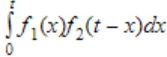Question 61

In a permutation a1...an of n distinct integers, an inversion is a pair (ai, aj) such that i < j and ai > aj.

If all permutations are equally likely, what is the expected number of inversions in a randomly chosen permutation of 1...n ?

 A n(n-1)/2 B n(n-1)/4 C n(n+1)/4 D 2n[log2n]
Algorithms        Sorting
Question 61 Explanation:
Probability of inverse (ai, aj(i Probability of expected no. of inversions = (1/2) × (n(n-1)/2) = n(n-1)/4
 Question 62

In a permutation a1...an of n distinct integers, an inversion is a pair (ai, aj) such that ii > aj.

What would be the worst case time complexity of the Insertion Sort algorithm, if the inputs are restricted to permutations of 1...n with at most n inversions?

 A Θ(n2) B Θ(n log n) C Θ(n1.5) D Θ(n)
Algorithms        Sorting
Question 62 Explanation:
Here the inputs are to be restricted to 1...n with atmost 'n' inversions. Then the worst case time complexity of inversion sort reduces to Θ(n).
 Question 63

A data structure is required for storing a set of integers such that each of the following operations can be done in O(log n) time, where n is the number of elements in the set.

I. Deletion of the smallest element
II. Insertion of an element if it is not already present in the set

Which of the following data structures can be used for this purpose?

 A A heap can be used but not a balanced binary search tree B A balanced binary search tree can be used but not a heap C Both balanced binary search tree and heap can be used D Neither balanced binary search tree nor heap can be used
Data-Structures       Tree
Question 63 Explanation:
→ In heap deletion takes O(log n).
Insertion of an element takes O(n).
→ In balanced primary tree deletion takes O(log n).
Insertion also takes O(log n).
 Question 64

Let S be a stack of size n≥1. Starting with the empty stack, suppose we push the first n natural numbers in sequence, and then perform n pop operations. Assume that Push and pop operation take X seconds each, and Y seconds elapse between the end of one such stack operation and the start of the next operation. For m≥1, define the stack-life of m as the time elapsed from the end of Push(m) to the start of the pop operation that removes m from S. The average stack-life of an element of this stack is

 A n(X + Y) B 3Y + 2X C n(X + Y) - X D Y + 2X
Data-Structures       Stacks
Question 64 Explanation:
Life time of last element present in the stack = Y
(After push into stack then immediately popped)
Life time of (n-1) element = Y + X + Y + X + Y = 2X + 3Y
Life time of (n-2) element = (n-1) + 2X + 2Y = 2X + 3Y + 2X + 2Y = 4X + 5Y
Life time of 1's element = 2(n-1)X + (2n-1)Y
Life time of all elements is ⇒
2X(1+2+3+...+n-1)+Y(1+3+5+...+(2n-1))
⇒ 2X(n(n-1) /2) +Y((n/2)(1+2n-1))
⇒ n(n(X+Y)-X))
Avg. of n numbers = n(n(X+Y)-X)/n = n(X+Y)-X
 Question 65

Consider the following 2-3-4 tree (i.e., B-tree with a minimum degree of two) in which each data item is a letter. The usual alphabetical ordering of letters is used in constructing the tree.What is the result of inserting G in the above tree?

 A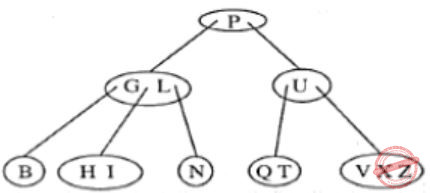BCD None of the above
Data-Structures       Trees
Question 65 Explanation:
Given Tree isInsert G at root level:Question 66

The cube root of a natural number n is defined as the largest natural number m such that m3≤n. The complexity of computing the cube root of n (n is represented in binary notation) is:

 A O(n) but not O(n0.5) B O(n0.5 but not O((log n)k) for any constant k>0 C O((log n)k) for some constant k>0, but not O((log log n)m) for any constant m>0 D O((log log n)k) for some constant k>0.5, but not O((log log n)0.5)
Algorithms        Time-Complexity
Question 66 Explanation:
Time complexity to search using binary search is O(log n). The cube root involves to search again O(log n) times in worst case. So time taken to find cube root is O(log2n) ... (I)
The time complexity is never less than O(log n) because to represent a binary search will takes O(log n) ... (II)
From (I), option A and B False
From (II), option D False.
 Question 67

Let G = (V, E) be an undirected graph with a subgraph G1 = (V1, E1). Weights are assigned to edges of G as follows: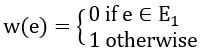A single-source shortest path algorithm is executed on the weighted graph (V,E,w) with an arbitrary vertex ν1 of V1 as the source. Which of the following can always be inferred from the path costs computed?

 A The number of edges in the shortest paths from v1 to all vertices of G B G1 is connected C V1 forms a clique in G D G1 is a tree
Algorithms Shortest-Path
Question 67 Explanation:
Calculate the minimum weight of the graph by using single source shortest path, if the weight of the graph is 0 then G is Connected, then that is the shortest path. In case the weight of the graph is not 0 then the graph G is disconnected.
 Question 68

What is the weight of a minimum spanning tree of the following graph ?A 29 B 31 C 38 D 41
Algorithms        Minimum-Spanning-Tree
Question 68 Explanation:1+2+3+2+8+4+4+2+5 =31
 Question 69

The following are the starting and ending times of activities A, B, C, D, E, F, G and H respectively in chronological order: "asbscsaedsceesfsbedegseefehsgehe" Here, xs denotes the starting time and xe denotes the ending time of activity X. We need to schedule the activities in a set of rooms available to us. An activity can be scheduled in a room only if the room is reserved for the activity for its entire duration. What is the minimum number of rooms required?

 A 3 B 4 C 5 D 6
Algorithms        Greedy-Algorit
Question 69 Explanation:
Given order is “as bs cs ae ds ce es fs be de gs ee fe hs ge he
Where xs→Starting time, xe→ Ending time.
R1→for a
R2→for b
R3→for c
ae, R1 is free
R1→for d
ce, R3 is free
R3→for e
R4→for f
be, R2 is free
de, R1 is free
R1→for g
ee, R3 is free
fe, R4 is free
R2→for h
ge, R1 is free
he, R2 is free
Here, total we used is: R1, R2, R3, R4.
Therefore, total no. of rooms we required = 4(minimum).
 Question 70

Let G = (V,E) be a directed graph with n vertices. A path from vi to vj in G is sequence of vertices (vi, vi+1, ......., vj) such that (vk, vk+1) ∈ E for all k in i through j-1. A simple path is a path in which no vertex appears more than once. Let A be an nxn array initialized as follow.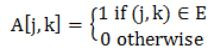Consider the following algorithm.

```for i = 1 to n
for j = 1 to n
for k = 1 to n
A [j,k] = max (A[j,k] (A[j,i] + A [i,k]);```

Which of the following statements is necessarily true for all j and k after terminal of the above algorithm?

 A A[j,k] ≤ n B If A[j,j] ≥ n-1, then G has a Hamiltonian cycle C If there exists a path from j to k, A[j,k] contains the longest path length from j to k D If there exists a path from j to k, every simple path from j to k contains at most A[j,k] edges
Algorithms        Graphs
Question 70 Explanation: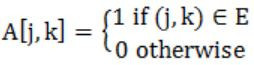Here, we have two functionalities i.e., from j to k, there exists a path then it results otherwise 0.
If there exist a path then it results
A[j,k] = max(A[j,k], A[j,i]+A[i,k])
i.e., if there exists a path from j to k, every simple path from j to k contains the atmost A[j,k] edges.
 Question 71

Consider the following logic program P

```A(x) <- B(x, y), C(y)
<- B(x,x) ```

Which of the following first order sentences is equivalent to P?

 A (∀x) [(∃y) [B(x,y) ∧ C(y)] ⇒ A(x)] ∧ ¬(∃x)[B(x,x)] B (∀x) [(∀y) [B(x,y) ∧ C(y)] ⇒ A(x)] ∧ ¬(∃x)[B(x,x)] C (∀x) [(∃y) [B(x,y) ∧ C(y)] ⇒ A(x)] ∨ ¬(∃x)[B(x,x)] D (∀x) [(∀y) [B(x,y) ∧ C(y)] ⇒ A(x)] ∧ (∃x)[B(x,x)]
Programming       Logic-Programming
Question 71 Explanation:
Note: This is not in gate syllabus. Please ignore this question.
 Question 72

The following resolution rule is used in logic programming.

`Derive clause (P ∨ Q) from clauses (P ∨ R), (Q ∨ ¬R) `

Which of the following statements related to this rule is FALSE?

 A ((P ∨ R) ∧ (Q ∨ ¬R)) ⇒ (P ∨ Q) is logically valid B (P ∨ Q) ⇒ ((P ∨ R) ∧ (Q ∨ ¬R)) is logically valid C (P ∨ Q) is satisfiable if and only if (P∨R) ∧ (Q∨¬R) is satisfiable D (P ∨ Q) ⇒ FALSE if and only if both P and Q are unsatisfiable
Engineering-Mathematics       Propositional-Logic
Question 72 Explanation:
(P ∨ Q) ⇒ ((P ∨ R) ∧ (Q ∨ ¬R))It is may be True (or) False depending on values. So this is not valid.
 Question 73

The following program fragment is written in a programming language that allows variables and does not allow nested declarations of functions.

```global int i = 100, j = 5;
void P(x)
{
int i = 10;
print(x + 10);
i = 200;
j = 20;
print(x);
}
main()
{
P(i + j);
}```

If the programming language uses static scoping and call by need parameter passing mechanism, the values printed by the above program are

 A 115, 220 B 25, 220 C 25, 15 D 115, 105
Programming       Parameter-Passing
Question 73 Explanation:
P(i+j)
P(100+5) = P(105)
→void P(105)
{
int i=10;
print (x+10);  ⇒ 105+10=115 prints
i=200;
j = 20;
print (x);  ⇒ x=105 prints
}
115, 105 prints
 Question 74

The following program fragment is written in a programming language that allows variables and does not allow nested declarations of functions.

```global int i = 100, j = 5;
void P(x)
{
int i = 10;
print(x + 10);
i = 200;
j = 20;
print(x);
}
main()
{
P(i + j);
}```

If the programming language uses dynamic scoping and call by name parameter passing mechanism, the values printed by the above program are:

 A 115, 220 B 25, 220 C 25, 15 D 115, 105
Programming       Parameter-Passing
Question 74 Explanation:
In dynamic,
In void P(x)
{  int i = 10;
print(x + 10);   ⇒ 105+10 = 115 printsprint (x);   ⇒ print x=220;
 Question 75

Consider the following class definitions in a hypothetical Object Oriented language that supports inheritance and uses dynamic binding. The language should not be assumed to be either Java or C++, though the syntax is similar.

```Class P {
void f(int i) {
print(i);
}
}

Class Q subclass of P {
void f(int i) {
print(2*i);
}
} ```

Now consider the following program fragment:

```Px = new Q();
Qy = new Q();
Pz = new Q();
x.f(1); ((P)y).f(1); z.f(1); ```

Here ((P)y) denotes a typecast of y to P. The output produced by executing the above program fragment will be

 A 1 2 1 B 2 1 1 C 2 1 2 D 2 2 2
Programming       Variable Binding
Question 75 Explanation:
Because of using dynamic binding it results a values such as 2 2 2.
Note: The given question is not in the present syllabus
 Question 76

Which of the following is NOT an advantage of using shared, dynamically linked libraries as opposed to using statically linked libraries?

 A Smaller sizes of executable files B Lesser overall page fault rate in the system C Faster program startup D Existing programs need not be re-linked to take advantage of newer versions of libraries
Compiler-Design       Run-Time-Environments
Question 76 Explanation:
Dynamic link libraries takes more time in program setup (in loading and linking phase to set up the global offset table and load and link the required libraries).
 Question 77

A uni-processor computer system only has two processes, both of which alternate 10ms CPU bursts with 90ms I/O bursts. Both the processes were created at nearly the same time. The I/O of both processes can proceed in parallel. Which of the following scheduling strategies will result in the least CPU utilization (over a long period of time) for this system?

 A First come first served scheduling B Shortest remaining time first scheduling C Static priority scheduling with different priorities for the two processes D Round robin scheduling with a time quantum of 5 ms
Operating-Systems       Process-Scheduling
Question 77 Explanation:
We have two processes P and Q. These process have 10ms CPU burst time and 90ms I/O bursts.
(i) FCFS:
0-10: Process 1 can execute
10-20: Process 2 can execute
100-110: Process 1 Terminate
110-120: Process 2 Terminate
CPU utilization = 20/100 [In every 100ms it utilizes]
=20%
(ii) SRTF: can process P and Q same as FCFS
then CPU utilization = 20%
(iii) Round robin: with TQ-5
0-5: Process P1
5-10: Process P2
10-15: Process P1
15-20: Process P2
105-110: Process P1
110-115: Process P2
CPU utilization = 20/105 = 19.5
 Question 78

A processor uses 2-level page tables for virtual to physical address translation. Page tables for both levels are stored in the main memory. Virtual and physical addresses are both 32 bits wide. The memory is byte addressable. For virtual to physical address translation, the 10 most significant bits of the virtual address are used as index into the first level page table while the next 10 bits are used as index into the second level page table. The 12 least significant bits of the virtual address are used as offset within the page. Assume that the page table entries in both levels of page tables are 4 bytes wide. Further, the processor has a translation look-aside buffer (TLB), with a hit rate of 96%. The TLB caches recently used virtual page numbers and the corresponding physical page numbers. The processor also has a physically addressed cache with a hit rate of 90%. Main memory access time is 10 ns, cache access time is 1 ns, and TLB access time is also 1 ns.

Assuming that no page faults occur, the average time taken to access a virtual address is approximately (to the nearest 0.5 ns)

 A 1.5 ns B 2 ns C 3 ns D 4 ns
Operating-Systems       Virtual Memory
Question 78 Explanation:
The possibilities are = (TLB Hit * Cache Hit) + (TLB Hit * Cache Miss)
(TLB Miss * Cache Hit) + (TLB Miss * Cache Miss)
= (0.96*0.9*2)+(0.96*0.1+12)
(0.04*0.9*2)+(0.04*0.1*32)
= 3.8
= 4 (approximately)
 Question 79

A processor uses 2-level page tables for virtual to physical address translation. Page tables for both levels are stored in the main memory. Virtual and physical addresses are both 32 bits wide. The memory is byte addressable. For virtual to physical address translation, the 10 most significant bits of the virtual address are used as index into the first level page table while the next 10 bits are used as index into the second level page table. The 12 least significant bits of the virtual address are used as offset within the page. Assume that the page table entries in both levels of page tables are 4 bytes wide. Further, the processor has a translation look-aside buffer (TLB), with a hit rate of 96%. The TLB caches recently used virtual page numbers and the corresponding physical page numbers. The processor also has a physically addressed cache with a hit rate of 90%. Main memory access time is 10 ns, cache access time is 1 ns, and TLB access time is also 1 ns.

Suppose a process has only the following pages in its virtual address space: two contiguous code pages starting at virtual address 0x00000000, two contiguous data pages starting at virtual address 0×00400000, and a stack page starting at virtual address 0×FFFFF000. The amount of memory required for storing the page tables of this process is:

 A 8 KB B 12 KB C 16 KB D 20 KB
Operating-Systems       Virtual Memory
Question 79 Explanation:
Breakup of given addresses into bit form:-
32bits are broken up as 10bits (L2) | 10bits (L1) | 12bits (offset)
First code page:
0x00000000 = 0000 0000 00 | 00 0000 0000 | 0000 0000 0000
So next code page will start from
0x00001000 = 0000 0000 00 | 00 0000 0001 | 0000 0000 0000
First data page:
0x00400000 = 0000 0000 01 | 00 0000 0000 | 0000 0000 0000
So next data page will start from
0x00401000 = 0000 0000 01 | 00 0000 0001 | 0000 0000 0000
Only one stack page:
0xFFFFF000 = 1111 1111 11 | 11 1111 1111 | 0000 0000 0000
Now, for second level page table, we will just require 1 Page
which will contain following 3 distinct entries i.e. 0000 0000 00, 0000 0000 01, 1111 1111 11.
Now, for each of these distinct entries, we will have 1-1 page in Level-1.
Hence, we will have in total 4 pages and page size = 212 = 4KB.
Therefore, Memory required to store page table = 4*4KB = 16KB.
 Question 80

Suppose we want to synchronize two concurrent processes P and Q using binary semaphores S and T. The code for the processes P and Q is shown below.

```Process P:
while (1) {
W:
print '0';
print '0';
X:
}

Process Q:
while (1) {
Y:
print '1';
print '1';
Z:
}```

Synchronization statements can be inserted only at points W, X, Y and Z.

Which of the following will always lead to an output staring with '001100110011'?

 A P(S) at W, V(S) at X, P(T) at Y, V(T) at Z, S and T initially 1 B P(S) at W, V(T) at X, P(T) at Y, V(S) at Z, S initially 1, and T initially 0 C P(S) at W, V(T) at X, P(T) at Y, V(S) at Z, S and T initially 1 D P(S) at W, V(S) at X, P(T) at Y, V(T) at Z, S initially 1, and T initially 0
Operating-Systems       Process-Synchronization
Question 80 Explanation:
Option B is correct.
Process P1 will be executed first and then Process P2 can be executed next.
At the process P: W=P(S)
X=V(T)
At the process Q: Y=P(T)
Z=V(S)
Here, S=1, T=0 then the process P executes first and then Q, and both can run on process alternate way start with P.
 Question 81

Suppose we want to synchronize two concurrent processes P and Q using binary semaphores S and T. The code for the processes P and Q is shown below.

```Process P:
while (1) {
W:
print '0';
print '0';
X:
}

Process Q:
while (1) {
Y:
print '1';
print '1';
Z:
}```

Synchronization statements can be inserted only at points W, X, Y and Z.

Which of the following will ensure that the output string never contains a substring of the form 01n0 or 10n1 where n is odd?

 A P(S) at W, V(S) at X, P(T) at Y, V(T) at Z, S and T initially 1 B P(S) at W, V(T) at X, P(T) at Y, V(S) at Z, S and T initially 1 C P(S) at W, V(S) at X, P(S) at Y, V(S) at Z, S initially 1 D V(S) at W, V(T) at X, P(S) at Y, P(T) at Z, S and T initially 1
Operating-Systems       Process-Synchronization
Question 81 Explanation:
Here to print the required output only one semaphore is enough, if we initialize two at a time then they will run concurrently and leads the processing error.
 Question 82

The subnet mask for a particular network is 255.255.31.0. Which of the following pairs of IP addresses could belong to this network?

 A 172.57.88.62 and 172.56.87.233 B 10.35.28.2 and 10.35.29.4 C 191.203.31.87 and 191.234.31.88 D 128.8.129.43 and 128.8.161.55
Computer-Networks       IP-Address
Question 82 Explanation:
To find whether hosts belong to same network or not , we have to find their net id, if net id is same then hosts belong to same network and net id can be find by ANDing subnet mask and IP address.
128.8.129.43 (Bitwise AND) 255.255.31.0 = 128.8.1.0
128.8.161.55 (Bitwise AND) 255.255.31.0 = 128.8.1.0
 Question 83

A 2 km long broadcast LAN has 107 bps bandwidth and uses CSMA/CD. The signal travels along the wire at 2×108 m/s. What is the minimum packet size that can be used on this network?

 A 50 bytes B 100 bytes C 200 bytes D None of the above
Computer-Networks       Ethernet
Question 83 Explanation:
Minimum packet size for a CSMA/CD LAN is the frame which cover whole RTT(round trip time). i.e. Tt = 2Tp
d= 2 km = 2 x 103 m, v = 2 x 108 m/s, B= 107
Tp = d / v = 2 x 103 /(2 x 108 ) seconds = 10-5 seconds
Let L bits be minimum size of frame, then Tt = t L / B = L / 107 seconds
Now, Tt = 2Tp
L/107 = 2 x 10-5 = 200 bits = (200 / 8) bytes = 25 bytes
 Question 84

Host A is sending data to host B over a full duplex link. A and B are using the sliding window protocol for flow control. The send and receive window sizes are 5 packets each. Data packets (sent only from A to B) are all 1000 bytes long and the transmission time for such a packet is 50 µs. Acknowledgement packets (sent only from B to A) are very small and require negligible transmission time. The propagation delay over the link is 200 µs. What is the maximum achievable throughput in this communication?

 A 7.69 × 106 bps B 11.11 × 106 bps C 12.33 × 106 bps D 15.00 × 106 bps
Computer-Networks       Sliding-Window-Protocol
Question 84 Explanation:
Given, Tt = 50 μs, Tp = 200 μs, L = 1000 bytes, N = 5,
Transmission rate , Tt = L / B.W
Therefore, B.W. = L / Tt = 1000 bytes/ 50 μs = 8000 bits / 50 μs=160 Mbps
Efficiency = N / 1 + 2a, where a = Tp / Tt
Efficiency = 5 * 50 / (50+400) = 250/450 = 5/9
Maximum achievable throughput = Efficiency * B.W = (5/9)*160 Mbps = 88.88 Mbps = = 11.11 x 106 bytes per second
*Actual option should be in bytes per second.
 Question 85

Consider the following functional dependencies in a database:

```  Data_of_Birth → Age
Age → Eligibility
Name → Roll_number
Roll_number → Name
Course_number → Course_name
Course_number → Instructor
(Roll_number, Course_number) → Grade ```

The relation (Roll_number, Name, Date_of_birth, Age) is:

 A in second normal form but not in third normal form B in third normal form but not in BCNF C in BCNF D in none of the above
Database-Management-System       Normalization
Question 85 Explanation:
Three FD's are valid from the above set of FD's for the given relation.
Date_of_Birth → Age
Name → Roll_number
Roll_number → Name
Candidate keys for the above are:
(Date_of_Birth, Name) and (Date_of_Birth, Roll_number)
Clearly, there is a partial dependency,
Date_of_Birth → Age
So, it is only in 1NF.
 Question 86

Consider the set of relations shown below and the SQL query that follows.

```  Students: (Roll_number, Name, Date_of_birth)
Courses: (Course number, Course_name, Instructor)
Grades: (Roll_number, Course_number, Grade)
select distinct Name
from Students, Courses, Grades
where Students. Roll_number = Grades.Roll_number
and Courses.Instructor = Korth
and Courses.Course_number = Grades.Course_number
and Grades.grade = A ```

Which of the following sets is computed by the above query?

 A Names of students who have got an A grade in all courses taught by Korth B Names of students who have got an A grade in all courses C Names of students who have got an A grade in at least one of the courses taught by Korth D in none of the above
Database-Management-System       SQL
Question 86 Explanation:
The query results a names of students who got an A grade in at least one of the courses taught by korth.
 Question 87

Consider three data items D1, D2 and D3 and the following execution schedule of transactions T1, T2 and T3. In the diagram, R(D) and W(D) denote the actions reading and writing the data item D respectively.Which of the following statements is correct?

 A The schedule is serializable as T2; T3; T1 B The schedule is serializable as T2; T1; T3 C The schedule is serializable as T3; T2; T1 D The schedule is not serializable
Database-Management-System       Transactions
Question 87 Explanation:
Precedence graph:Cycle formed so not serializable.
 Question 88

In the following C program fragment, j, k n and TwoLog_n are interger variables, and A is an array of integers. The variable n is initialized to an integer ≥3, and TwoLog_n is initialized to the value of 2*⌈log2(n)⌉

```   for (k = 3; k < = n; k++)
A[k] = 0;
for (k = 2; k < = TwoLog_n; k++)
for (j = k + 1; j < = n; j++)
A[j] = A[j] || (j%k);
for (j = 3; j < = n; j++)
if (!A[j]) printf("%d", j); ```

The set of numbers printed by this program fragment is

 A {m|m ≤ n, (∃i)[m=i!]} B {m|m ≤ n, (∃i)[m=i2]} C {m|m ≤ n, m is prime} D { }
Programming       C-Programming
Question 88 Explanation:
Take n=4, so Two log_n=4
Now Trace the code,
for (k=3; k<=n; k++)
A[k]=0; // A=0
A=0
for (k=2; k<=Two log_n; k++)
for(j=k+1; j<=n; j++)
A[j] = A[j] // (j%k); // A = 0 // I=1
A = 0 // I=1
for (j=3; j<=n; j++)
if (!A[j]) printf("%d", j);
// if (!1) means if (0), so printf will never execute
Hence, Option (D) is the answer.
 Question 89

Consider the C program shown below.

```#include
#define print(x) printf("%d", x)
int x;
void Q(int z)
{
z += x;
print(z);
}
void P(int *y)
{
int x = *y + 2;
Q(x);
*y = x - 1;
print(x);
}
main(void)
{
x = 5;
P(&x);
print(x);
} ```

The output of this program is

 A 12 7 6 B 22 12 11 C 14 6 6 D 7 6 6
Programming       C-Programming
Question 89 Explanation:
In main function x=5; it is global array
p(&x) it goes to P( ) function
y=5
x=5+2=7;
Q(x)
z=7
z=7+5=12(Print+z→I)
comes to P( )
*y=7-1=6
x=7(Print x→II)
comes to main ( ),
print x=*y=6 (print x→III)
Output: 12 7 6
 Question 90

Consider the function f defined below.

```    struct item {
int data;
struct item * next;
};
int f(struct item *p) {
return ((p == NULL) || (p->next == NULL) ||
((P->data <= p->next->data) &&
f(p->next)));
} ```

For a given linked list p, the function f returns 1 if and only if

 A the list is empty or has exactly one element B the elements in the list are sorted in non-decreasing order of data value C the elements in the list are sorted in non-increasing order of data value D not all elements in the list have the same data value
Data-Structures       Linked-List
Question 90 Explanation:
It return a value '1' when the elements in the list are presented in sorted order and non-decreasing order of data value.
There are 90 questions to complete.
PHP Code Snippets Powered By : XYZScripts.com
error: Content is protected !!